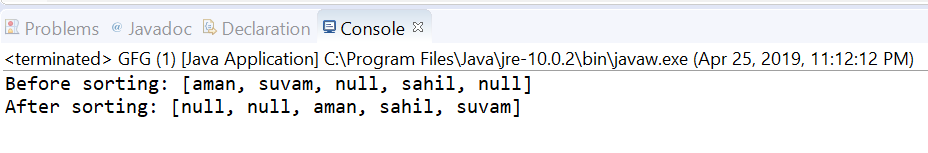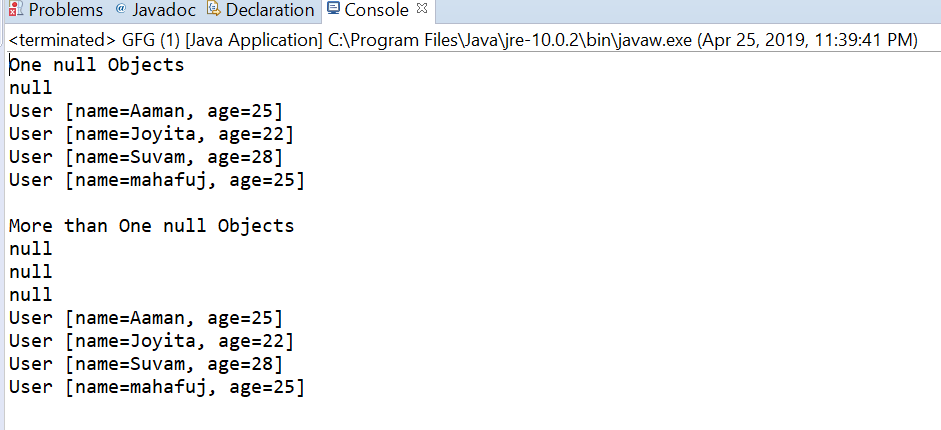# Comparator nullsFirst() method in Java with examples

The nullsFirst(java.util.Comparator) method returns comparator that is a null-friendly comparator and considers null values to be less than non-null. The null first operates by the following logic:

1. The null element is considered to be less than non-null.
2. When both elements are null, then they are considered equal.
3. When both elements are non-null, the specified Comparator determines the order.
4. If the specified comparator is null, then the returned comparator considers all non-null elements equal.
5. The returned comparator is serializable if the specified comparator is serializable.

Syntax:

```static <T> Comparator<T> nullsFirst(Comparator<? super T> comparator)
```

Parameters: This method accepts a single parameter comparator which is a Comparator for comparing non-null values

Return value: This method returns a comparator that considers null to be less than non-null and compares non-null objects with the supplied Comparator.

Below programs illustrate nullsFirst(java.util.Comparator) method:
Program 1:

 `// Java program to demonstrate ` `// Comparator.nullsFirst(java.util.Comparator) method ` ` `  `import` `java.util.Arrays; ` `import` `java.util.Comparator; ` ` `  `public` `class` `GFG { ` ` `  `    ``public` `static` `void` `main(String[] args) ` `    ``{ ` ` `  `        ``// Create a collection of an array ` `        ``// of names also contain nulls ` `        ``String[] strings = { ``"aman"``, ``"suvam"``, ` `                             ``null``, ``"sahil"``, ` `                             ``null` `}; ` ` `  `        ``// print the array ` `        ``System.out.println(``"Before sorting: "` `                           ``+ Arrays.toString(strings)); ` ` `  `        ``// apply nullsFirst method ` `        ``// and sort the array ` `        ``Arrays.sort(strings, ` `                    ``Comparator.nullsFirst( ` `                        ``Comparator.naturalOrder())); ` ` `  `        ``// print the array ` `        ``System.out.println(``"After sorting: "` `                           ``+ Arrays.toString(strings)); ` `    ``} ` `} `

The output printed on console of IDE is shown below.
Output:Program 2:

 `// Java program to demonstrate ` `// Comparator.nullsFirst(java.util.Comparator) method ` ` `  `import` `java.util.Arrays; ` `import` `java.util.Collections; ` `import` `java.util.Comparator; ` `import` `java.util.List; ` `public` `class` `GFG { ` `    ``public` `static` `void` `main(String[] args) ` `    ``{ ` ` `  `        ``// Create some user objects ` `        ``User u1 = ``new` `User(``"Aaman"``, ``25``); ` `        ``User u2 = ``new` `User(``"Joyita"``, ``22``); ` `        ``User u3 = ``new` `User(``"Suvam"``, ``28``); ` `        ``User u4 = ``new` `User(``"mahafuj"``, ``25``); ` ` `  `        ``System.out.println(``"One null Objects"``); ` `        ``List list ` `            ``= Arrays.asList(u1, u2, u3, ``null``, u4); ` `        ``Collections.sort(list, ` `                         ``Comparator.nullsFirst( ` `                             ``Comparator.comparing( ` `                                 ``User::getName))); ` `        ``list.forEach(user -> System.out.println(user)); ` ` `  `        ``System.out.println(``"\nMore than One null Objects"``); ` `        ``list = Arrays.asList(u1, u4, ``null``, u2, u3, ``null``, ``null``); ` `        ``Collections.sort(list, ` `                         ``Comparator.nullsFirst( ` `                             ``Comparator.comparing( ` `                                 ``User::getName))); ` `        ``list.forEach(user -> System.out.println(user)); ` `    ``} ` `} ` ` `  `class` `User ``implements` `Comparable { ` `    ``public` `String name; ` `    ``public` `int` `age; ` ` `  `    ``public` `User(String name, ``int` `age) ` `    ``{ ` `        ``this``.name = name; ` `        ``this``.age = age; ` `    ``} ` ` `  `    ``public` `int` `compareTo(User u1) ` `    ``{ ` `        ``return` `name.compareTo(u1.name); ` `    ``} ` ` `  `    ``public` `String getName() ` `    ``{ ` `        ``return` `name; ` `    ``} ` ` `  `    ``public` `void` `setName(String name) ` `    ``{ ` `        ``this``.name = name; ` `    ``} ` ` `  `    ``public` `int` `getAge() ` `    ``{ ` `        ``return` `age; ` `    ``} ` ` `  `    ``public` `void` `setAge(``int` `age) ` `    ``{ ` `        ``this``.age = age; ` `    ``} ` ` `  `    ``@Override` `    ``public` `String toString() ` `    ``{ ` `        ``return` `"User [name="` `+ name + ``", age="` `+ age + ``"]"``; ` `    ``} ` `} `

The output printed on console is shown below.
Output:Attention reader! Don’t stop learning now. Get hold of all the important Java and Collections concepts with the Fundamentals of Java and Java Collections Course at a student-friendly price and become industry ready.

My Personal Notes arrow_drop_upCheck out this Author's contributed articles.

If you like GeeksforGeeks and would like to contribute, you can also write an article using contribute.geeksforgeeks.org or mail your article to contribute@geeksforgeeks.org. See your article appearing on the GeeksforGeeks main page and help other Geeks.

Please Improve this article if you find anything incorrect by clicking on the "Improve Article" button below.

Article Tags :
Practice Tags :

Be the First to upvote.

Please write to us at contribute@geeksforgeeks.org to report any issue with the above content.